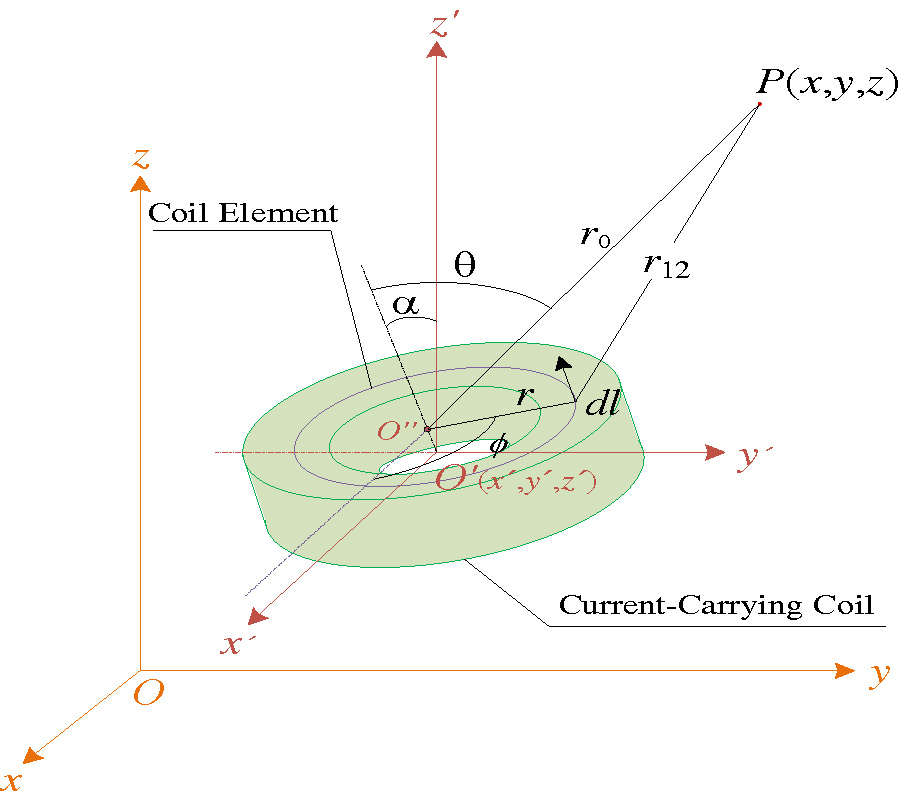# Vol. 101

Front:[PDF file] Back:[PDF file]
Latest Volume
All Volumes
All Issues
2021-02-07

#### Spatial Magnetic Field Calculations for Coreless Circular Coils with Rectangular Cross-Section of Arbitrary Turn Numbers

By Yiming Wang, Xu Xie, and Hengfeng Wang
Progress In Electromagnetics Research M, Vol. 101, 9-23, 2021
doi:10.2528/PIERM21010802

## Abstract

In a wireless magnetic induction communication system, the magnetic field distribution of the current-carrying coil affects the communication effect between the communication transceiver and receiver. In the study of magnetic field distribution, it was found that magnetic induction intensity and magnetic flux were important parameters to measure the effectiveness of communication. Aiming at the circular coils with rectangular cross-section of any turn numbers, this paper proposed an improved algorithm to calculate the magnetic induction intensity at any spatial position based on Biot-Savart law. At the same time, the calculation formula of the magnetic flux at the receiving point was also given. The coils were modeled and simulated with COMSOL software. The correctness of the improved algorithm was verified and compared with the traditional formula and simulation results, especially in the near field, which provided an important theoretical support for the further study of mutual inductance in the wireless magnetic induction communication system.

## Citation

Yiming Wang, Xu Xie, and Hengfeng Wang, "Spatial Magnetic Field Calculations for Coreless Circular Coils with Rectangular Cross-Section of Arbitrary Turn Numbers," Progress In Electromagnetics Research M, Vol. 101, 9-23, 2021.
doi:10.2528/PIERM21010802
http://www.jpier.org/PIERM/pier.php?paper=21010802

## References

1. Conway, J. T., "Trigonometric Integrals for the magnetic field of the coil of rectangular cross section," IEEE Transactions on Magnetics, Vol. 42, No. 5, 1538-1548, 2006.
doi:10.1109/TMAG.2006.871084

2. Ravaud, R., et al., "Cylindrical magnets and coils: Fields, forces, and inductances," IEEE Transactions on Magnetics, Vol. 46, No. 9, 3585-3590, 2010.
doi:10.1109/TMAG.2010.2049026

3. Guo, Z. Y. and D. J. Liu, "A numerical calculation method for spatial magnetic field of circular current," Science Technology and Engineering, Vol. 13, No. 29, 8715-8720, 2013.

4. Chen, X. W., et al., "Theoretical analysis and discussion on magnetic field of current-carrying coil and finite-length straight solenoid," College Physics, Vol. 38, No. 10, 23-27, 2019.

5. Chen, X. W., et al., "On Theoretical calculation and experimental discussion of magnetic field due to Helmholtz coil," Journal of Southwest China Normal University (Natural Science Edition), Vol. 45, No. 3, 40-45, 2020.

6. Wang, Y., "Analysis of axial magnetic field of coil coupler," Instrument Technology, No. 4, 14-16, 2020.

7. Guru, B. S. and H. R. Hiziroglu, Electromagnetic Field Theory Fundamentals, 2nd Ed., China Machine Press, Beijing, 2012.

8. Li, L., Antenna and Radio Wave Propagation, Science Press, Beijing, 2009.

9. Wang, Y. M., et al., "Calculation and modeling analysis of mutual inductance between coreless circular coils with rectangular cross section in arbitrary spatial position," Proceedings of IEEE 5th Information Technology and Mechatronics Engineering Conference, 1258-1267, Chongqing, China, 2020.

10. He, X. X., W. P. Ding, and J. X. Liu, Engineering Electromagnetic Field, Electronic Industry Press, Beijing, 2011.